﻿ PMC Research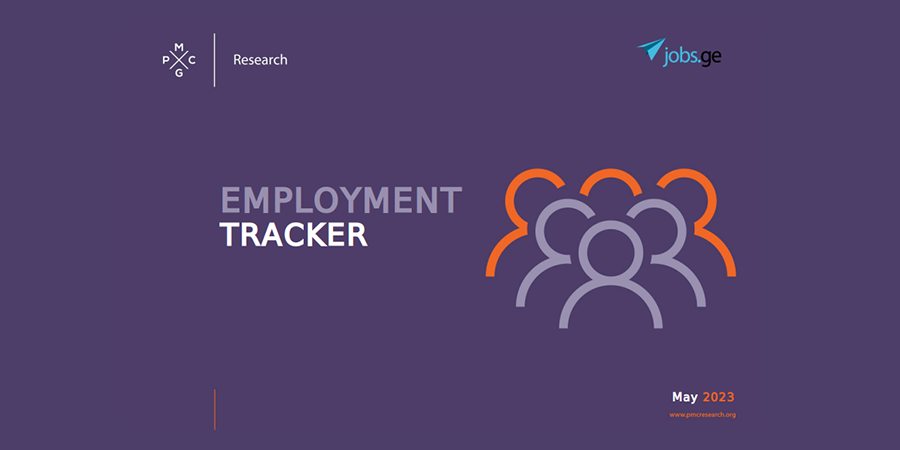Employment Tracker (May, 2023)
30-May-2023
In April 2023, the number of persons receiving a monthly salary increased by 3.7% compared to the corresponding period of 2022. In April 2023, the share of persons receiving a monthly salary of 2,400 GEL and more amounted to 21.8%, which was 5.7 percentage points more compared to the corresponding period of 2022. From November 2022 to April 2023, the total number of vacancies published on jobs.ge amounted to 38,330, which was 18.7% higher compared to the corresponding period of 2021- 2022.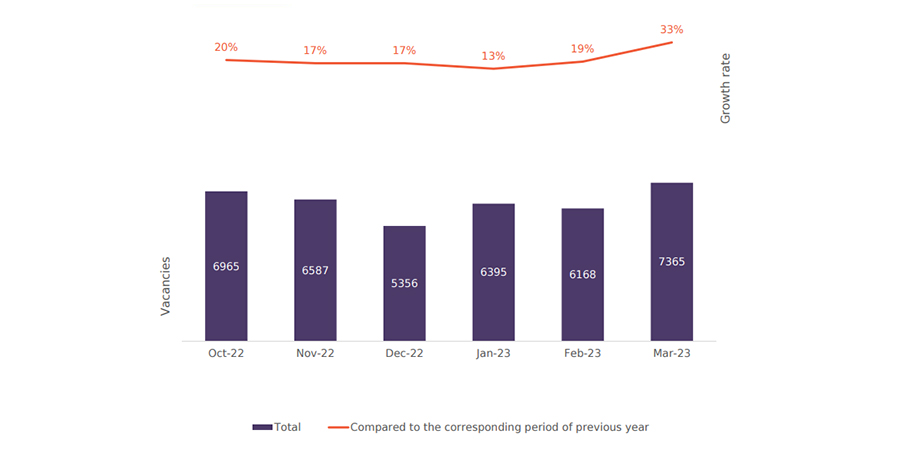Employment Tracker (April, 2023)
25-Apr-2023
In March 2023, the number of persons receiving a monthly salary increased by 3.9% compared to the corresponding period of 2022 and by 8.3% compared to the corresponding period of 2021. From October 2022 to March 2023, the total number of vacancies published on jobs.ge amounted to 38,836, which was 20.0% higher compared to the corresponding period of 2021- 2022. In March 2023, compared to February 2023, the total number of vacancies published on jobs.ge increased by 19.4%. From October 2022 to March 2023, a total of 7,047 vacancies were published in the field of finance, statistics, which was 42.5% higher than the corresponding period of 2021-2022 and 179.9% higher compared to the corresponding period of 2020-2021.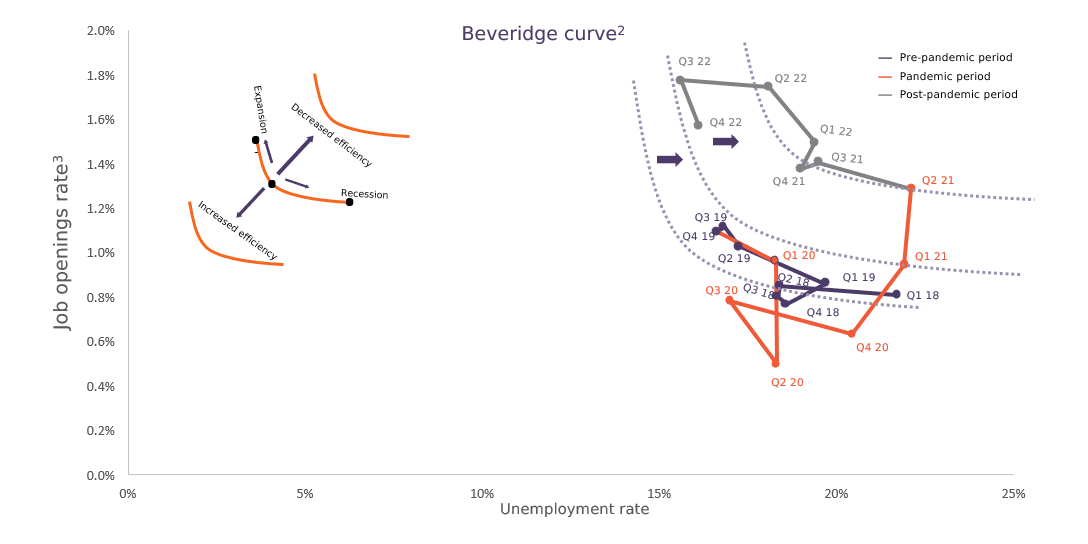Employment Tracker (March, 2023)
23-Mar-2023
In February 2023, the number of persons receiving a monthly salary increased by 4.9% compared to the corresponding period of 2022 and by 11.6% compared to the corresponding period of 2021. From September 2022 to February 2023, the total number of vacancies published on jobs.ge amounted to 39,565, which was 20.4% higher compared to the corresponding period of 2021-2022. In February 2023, compared to January 2023, the total number of vacancies published on jobs.ge decreased by 3.5%. From September 2022 to February 2023, a total of 7,065 vacancies were published in the field of finance, statistics, which was 40.6% higher than the corresponding period of 2021-2022 and 192.5% higher compared to the corresponding period of 2020-2021.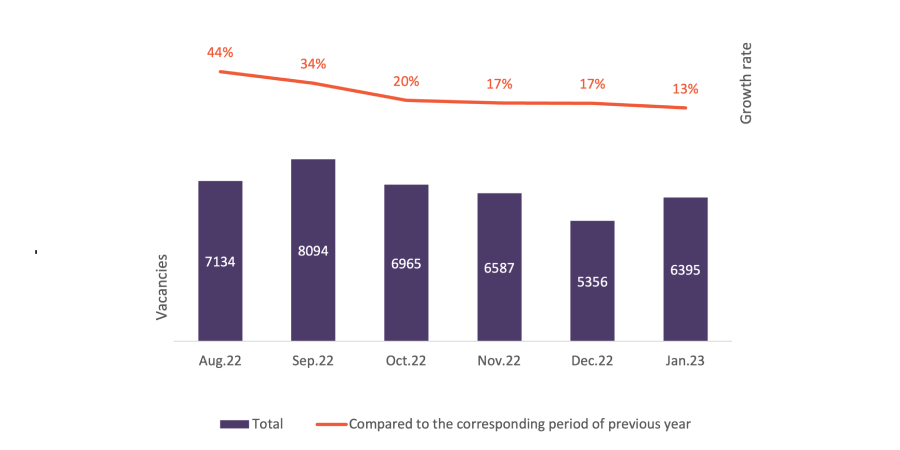Employment Tracker (February, 2023)
23-Feb-2023
In January 2023, the number of persons receiving a monthly salary increased by 2.5% compared to the corresponding period of 2022 and by 10.7% compared to the corresponding period of 2021. From August 2022 to January 2023, the total number of vacancies published on jobs.ge amounted to 40,531, which was 24.3% higher compared to the corresponding period of 2021-2022. In January 2023, compared to December 2022, the total number of vacancies published on jobs.ge increased by 19%. From August 2022 to January 2023, a total of 7,077 vacancies were published in the field of finance, statistics, which was 142.9% higher than the corresponding period of 2021-2022 and 297.2% higher compared to the corresponding period of 2020-2021.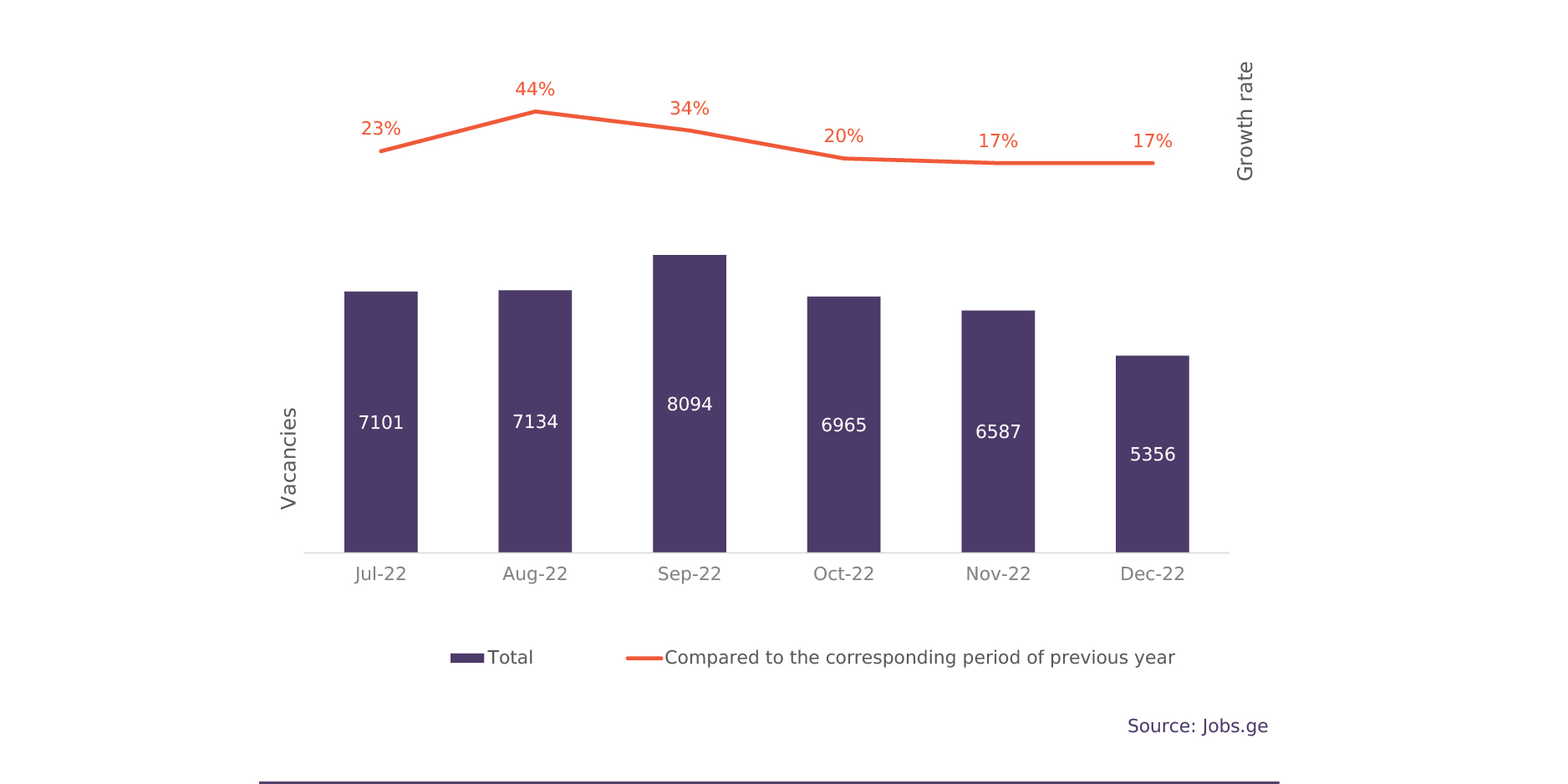Employment Tracker (January, 2023)
24-Jan-2023
In December 2022, the number of persons receiving a monthly salary increased by 2.9% compared to the corresponding period of 2021 and by 6.4% compared to the corresponding period of 2020. In 2022, from July to December, the total number of vacancies published on jobs.ge amounted to 41,237, which was 26% higher compared to the corresponding period of 2021 and 159.4% higher compared to the corresponding period of 2020. In December 2022, compared to November 2022, the total number of vacancies published on jobs.ge decreased by 19%. In 2022, from July to December, a total of 11,928 vacancies were published in the field of sales/procurement, which was 41% higher than the corresponding period of 2021 and 197% higher compared to the corresponding period of 2020.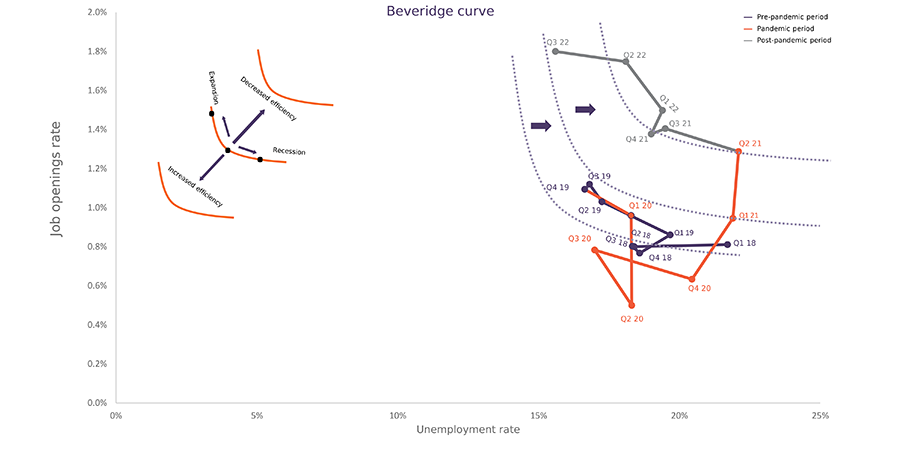Employment Tracker (December, 2022)
22-Dec-2022
In November 2022, the number of persons receiving a monthly salary increased by 1.4% compared to the corresponding period of 2021 and by 5.4% compared to the corresponding period of 2020. Overall, in Q4 of 2022, the BAG Employment Barometer amounted to 29.1 points, which is 1 point more than in Q3. In Q4 of the 2022 year, the Employment Barometer was positive for all sectors. Overall, in Q4 of 2022, according to the BAG Index Survey 35% of surveyed companies increased their number of employees, 7% recorded a decrease, and 58% reported no change. In 2022, from June to November, the total number of vacancies published on jobs.ge amounted to 43,323, which was 27.7% higher compared to the corresponding period of 2021 and 154.3% higher compared to the corresponding period of 2020. In 2022, from June to November, a total of 12,702 vacancies were published in the field of sales/procurement, which was 48% higher than the corresponding period of 2021 and 162% higher compared to the corresponding period of 2020. According to the Beveridge Curve, labor market efficiency decreased in the post-pandemic period (Q3 2022), compared to the pre-pandemic period (Q4 2019).  The latter trend could be attributed to different factors, including labor force migration, the flow of personnel to adjacent sectors, and increased wage expectations of job-seekers (i.e., the minimum wage that candidates are prepared to work for).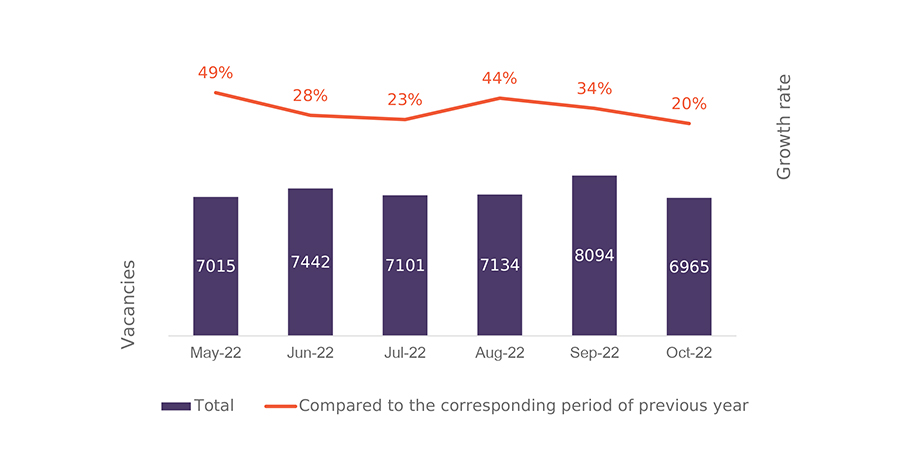Employment Tracker (November, 2022)
22-Nov-2022
In October 2022, the number of persons receiving a monthly salary decreased by 0.4% compared to the corresponding period of 2021 and increased by 3.7% compared to the corresponding period of 2020. In 2022, from May to October, the total number of vacancies published on jobs.ge amounted to 43,751, which was 32% higher compared to the corresponding period of 2021 and 164% higher compared to the corresponding period of 2020. In 2022, from May to October, a total of 12,962 vacancies were published in the field of sales/procurement, which was 58% higher than the corresponding period of 2021 and 209% higher compared to the corresponding period of 2020.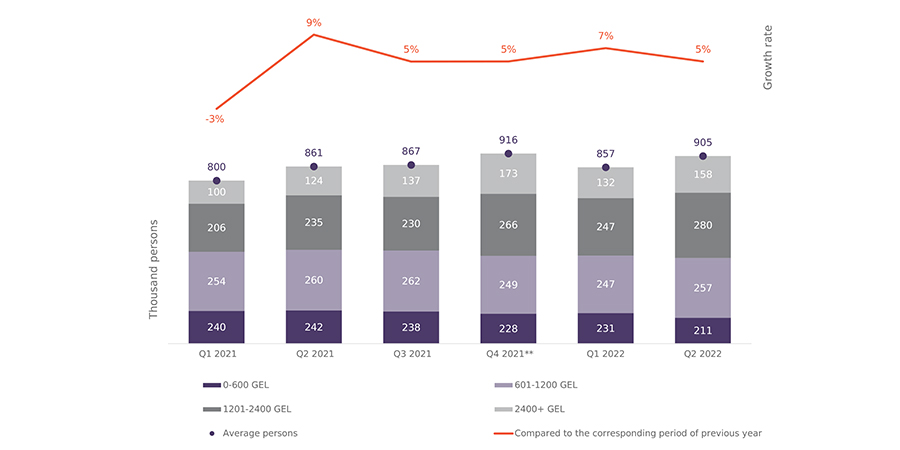Employment Tracker (October, 2022)
25-Oct-2022
In September 2022, the number of persons receiving a monthly salary increased by 3.5% compared to the corresponding period of 2021 and by 6.3% compared to the corresponding period of 2020. Overall, in Q2 of 2022, the BAG Employment Barometer amounted to 27.7 points, which is 2.8 points more than in Q1. In Q2 of 2022 year, the Employment Barometer was positive for all sectors. Overall, according to the BAG Index Survey, in Q2 of 2022, 32.1% of surveyed companies increased their number of employees, 6.3% recorded a decrease, and 61.6% reported no change. In 2022, from April to September, the total number of vacancies published on jobs.ge amounted to 42,523, which was 37% higher compared to the corresponding period of 2021 and 194% higher compared to the corresponding period of 2020. In 2022, from April to September, a total of 12,628 vacancies were published in the field of sales/procurement, which was 63% higher than the corresponding period of 2021 and 263% higher compared to the corresponding period of 2020.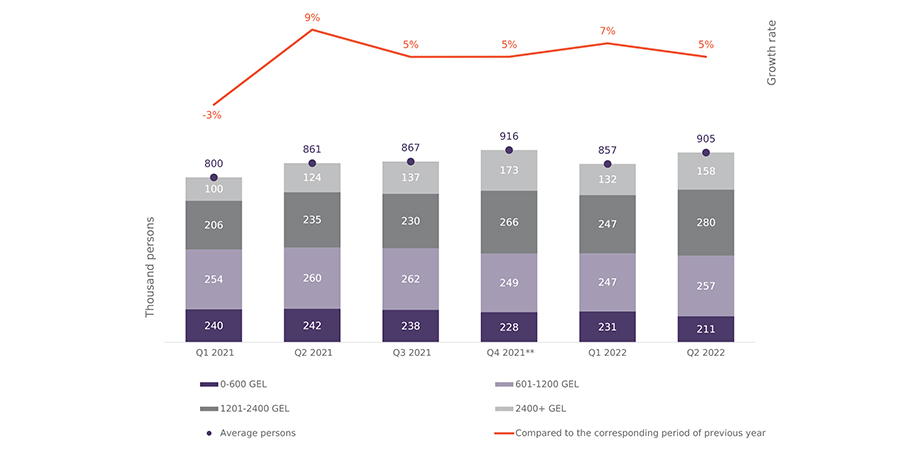Employment Tracker (September, 2022)
26-Sep-2022
In August 2022, the number of persons receiving a monthly salary increased by 3.5% compared to the corresponding period of 2021 and by 7.7% compared to the corresponding period of 2020. In 2022, from March to August, the total number of vacancies published on jobs.ge amounted to 39,984, which was 37% higher compared to the corresponding period of 2021 and 197% higher compared to the corresponding period of 2020. In 2022, from March to August, a total of 11,757 vacancies were published in the field of sales/procurement, which was 64% higher than the corresponding period of 2021 and 258% higher compared to the corresponding period of 2020.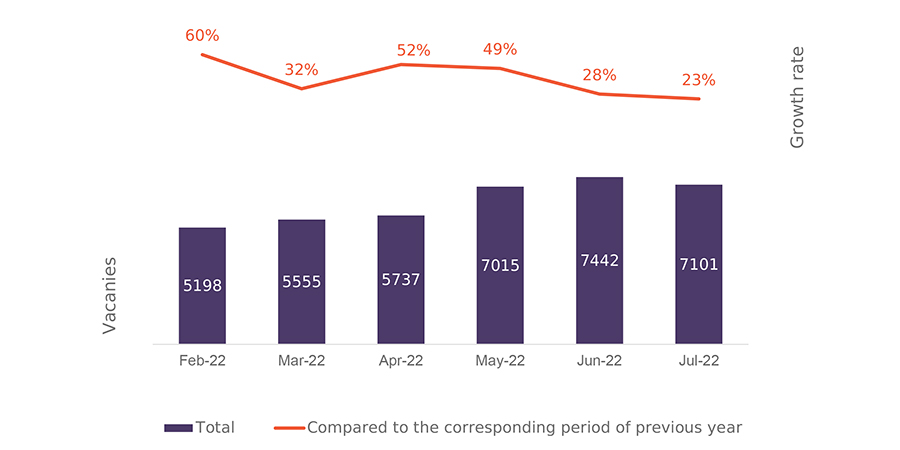Employment Tracker (August, 2022)
23-Aug-2022
In July 2022, the number of persons receiving a monthly salary increased by 1% compared to the corresponding period of 2021 and by 4.5% compared to the corresponding period of 2020. In 2022, from February to July, the total number of vacancies published on jobs.ge amounted to 38,048, which was 38% higher compared to the corresponding period of 2021 and 155% higher compared to the corresponding period of 2020. In 2022, from February to July, a total of 11,179 vacancies were published in the field of sales/procurement, which was 62% higher than the corresponding period of 2021 and 215% higher compared to the corresponding period of 2020.